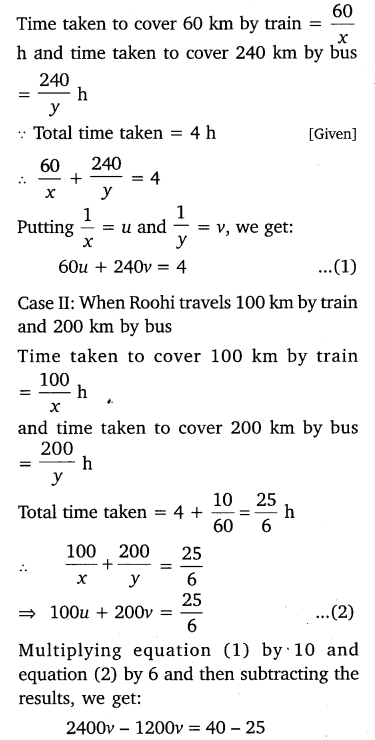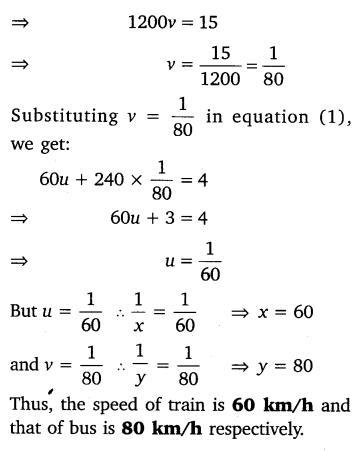# CLASS 10 MATH NCERT SOLUTIONS FOR CHAPTER – 3 PAIR OF LINEAR EQUATIONS IN TWO VARIABLES EX – 3.6

## Pair of Linear Equations in Two Variables

Question 1.
Solve the following pairs of equations by reducing them to a pair of linear equations: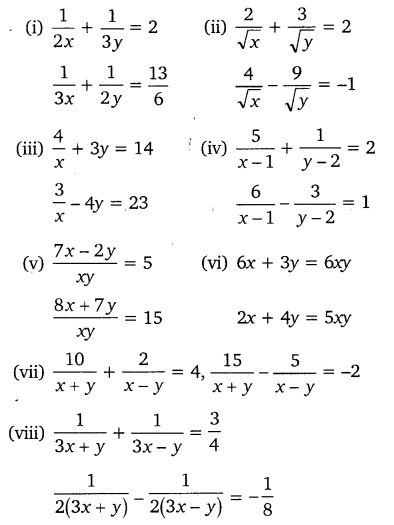Solution: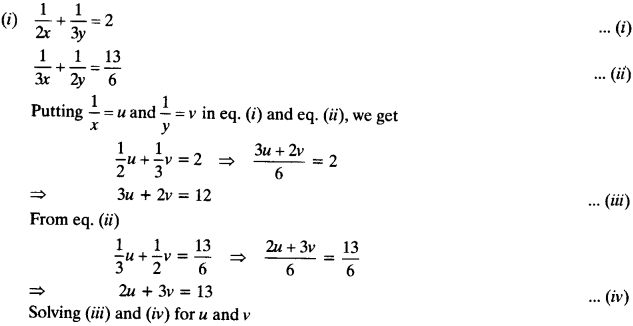By cross multiplication method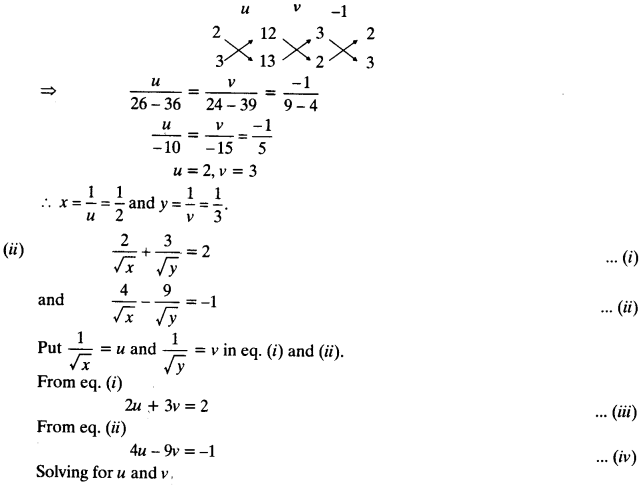By cross multiplication method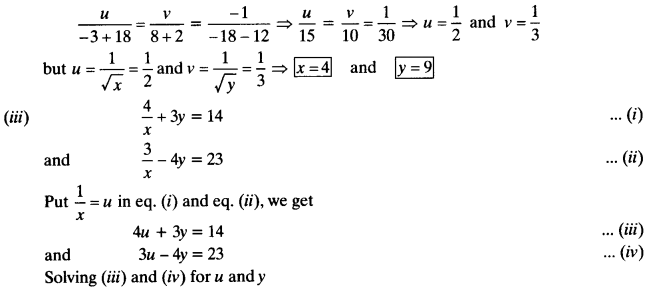By cross multiplication methodBy cross multiplication method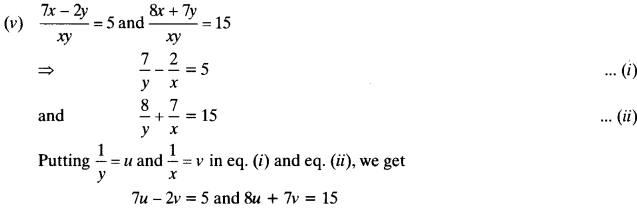solving for u and v by cross multiplication method: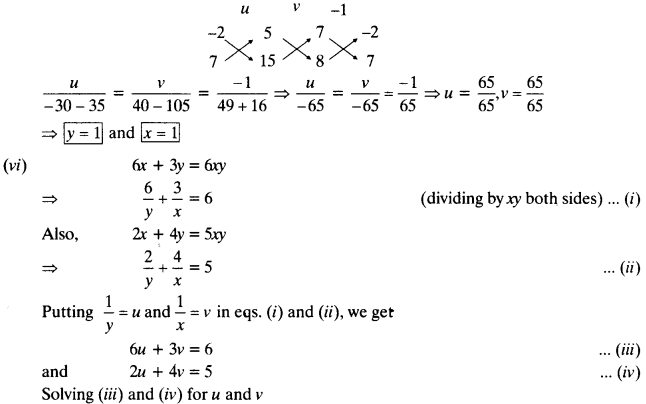By cross multiplication method: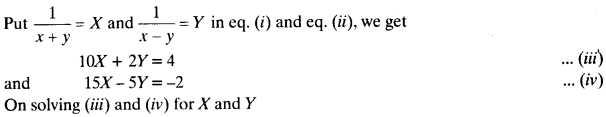By cross multiplication method: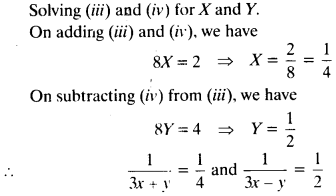Question 2.
Formulate the following problems as a pair of linear equations and hence find their solutions:

(i) Ritu can row downstream 20 km in hours and upstream 4 km in 2 hours. Find her speed of rowing in still water and the speed of the current.

(ii) 2 women and 5 men can together finish an embroidery work in 4 days, while 3 women and 6 men can finish it in 3 days. Find the time taken by 1 woman alone to finish the work, and also that taken by 1 man alone.

(iii) Roohi travels 300 km to her home partly by train and partly by bus. She takes 4 hours if she travels 60 km by train and the remaining by bus. If she travels 100 km by train and the remaining by bus, she takes 10 minutes longer. Find the speed of the train and the bus separately.

Solution:

(i) Let Ritu’s speed of rowing in still water be x km/h and speed of current be y km/h.
Speed of rowing in downstream = (x+y) km/h
and speed of rowing in upstream = (x-y) km/h
According to the question, we have:(ii) Let 1 woman can finish the work in x days  and 1 man can finish the work in y days.
Then 1 woman’s one day’s work =$\frac { 1 }{ x }$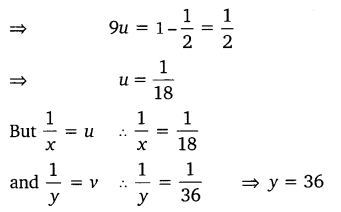Hence, one woman can finish the work in 18 days and one man can finish it in 36 days.

(iii) Let the speeds of the train and the bus be x km/h and y km/h respectively.
Case I: When Roohi travels 60 km by train and 240 km by bus